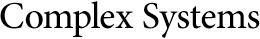## On the Structure of Multilayer Cellular Neural Networks: Complexity between Two Layers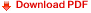Jung-Chao Ban*

Department of Applied Mathematics, National Dong Hwa University
Hualian 97401, Taiwan, ROC

Chih-Hung Chang

Department of Applied Mathematics, National University of Kaohsiung
Kaohsiung 81148, Taiwan, ROC
*jcban@mail.ndhu.edu.tw
Corresponding author: chchang@nuk.edu.tw

#### Abstract

Let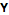be the solution space of an n-layer cellular neural network, and let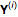and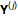be the hidden spaces, where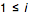,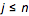. (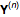is called the output space.) The classification and the existence of factor maps between two hidden spaces that reach the same topological entropies are investigated in . This paper elucidates the existence of factor maps between those hidden spaces carrying distinct topological entropies. For either case, the Hausdorff dimensions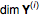and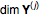can be calculated. Furthermore, the dimensions ofand) are related by the factor map between them.

https://doi.org/10.25088/ComplexSystems.24.4.311